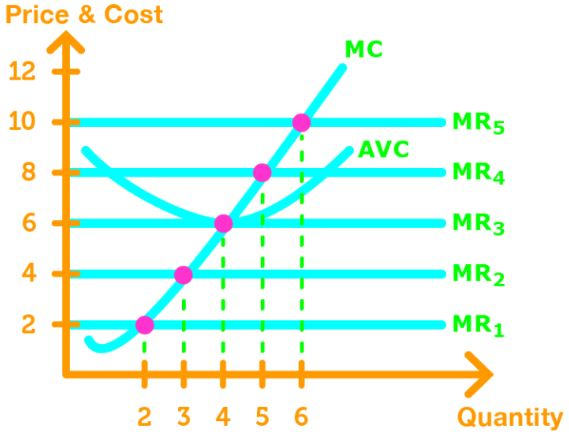# Perfect competition firm's output decisions#### Everything You Need in One Place

Homework problems? Exam preparation? Trying to grasp a concept or just brushing up the basics? Our extensive help & practice library have got you covered.#### Learn and Practice With Ease

Our proven video lessons ease you through problems quickly, and you get tonnes of friendly practice on questions that trip students up on tests and finals.#### Instant and Unlimited Help

Our personalized learning platform enables you to instantly find the exact walkthrough to your specific type of question. Activate unlimited help now!##### Intros
###### Lessons
1. Firm's Output Decisions Overview:
2. Total Revenue & Total Cost Curves
• 2 ways for markets to maximize profit
• Low output level $\,$$\,$ economic loss
• High output level $\,$$\,$ economic loss
• Intermediate output level $\,$$\,$ economic profits
• Find the output with the biggest gap
3. MC = MR
• Profit maximized when MC = MR
• MR > MC, economic profit $\, \uparrow \,$ when output $\, \uparrow \,$
• MR < MC, economic profit $\, \downarrow \,$ when output $\, \uparrow \,$
• MR = MC, economic profit $\, \downarrow \,$ when output $\, \uparrow \,$ or $\, \downarrow \,$
4. A Firm's Decision to Shutdown
• Calculating Economic Loss
• Shutdown $\,$$\,$ Firm must cover TFC
• Open $\,$$\,$ Firm must cover TFC & TVC
• Open when AVC < p, Close when AVC > p
• Shutdown Point when AVC = p
5. Firm's Supply Curve
• Market price varies, MR varies
• How much output firms make when price varies
##### Examples
###### Lessons
1. Maximizing Economic Profit, Analyzing Firm Operation, & Graphing Firm Supply Curves
Use the following table to answer the questions
 Output Total Cost 0 5 1 10 2 13 3 18 4 25 5 34
1. Calculate the profit-maximizing output and economic profit if the market price is $7. 2. What is the shutdown point? 3. What is the economic loss if the firm shuts down temporarily? 2. Use the following table to answer the questions  Output Total Cost 0 5 1 10 2 13 3 18 4 25 5 34 1. Calculate the profit-maximizing output and economic profit if the market price is$5.
2. Should the firm shut down or operate?
3. Consider the following graphFind the profit-maximizing output & economic profit when the market price is:
1. $10 2.$20
4. Consider the following graphGraph the firm's supply curve.
###### Topic Notes

There are 2 ways for firms to maximize profits:
1. Examine the Total Revenue & Total Cost curves
2. Find the output of when MR = MC

Total Revenue & Total Cost Curves

When examining the total revenue and total cost curves, we want to see which outputs give economic loss, and economic profit.When TR < TC, when we have an economic loss. This happens at low and high levels of output production.

When TR = TC, we gain no profit and no loss. Then happens when the curves intersect.

When TR > TC, we gain economic profit. This happens at intermediate levels of output production.

Goal: Find an intermediate level of output that maximizes profit. In other words, find the biggest gap between TR & TC!

Note: We can also graph the economic profit curve (P = TR - TC), and find the point with the highest economic profit!MC = MR

Recall that the marginal revenue (firms demand curve) is horizontal.

In addition, the marginal cost decreases as output increases in the beginning, and then increases afterwards.

Therefore, we get the following graph.If MR > MC, economic profit increases as output increases.

If MR < MC, economic profit decreases as output increases.

If MR < MC, economic profit does not change if output increases or decreases. Profit is maximized here.

A Firms Decision to Shutdown

To see if a firm should stay open or shut down, we need to know how to calculate the economic loss of a firm.

Economic Loss = TFC + TVC - TR
= TFC + (AVC x q) - (p x q)
= TFC + (AVC - p) x q

When the firm decides to shutdown, then q = 0, and the economic loss is TFC. If the firm stay open, then q > 0, and the firm would have to pay both TFC and TVC.

The firm should stay open when AVC < p .

The firm should shut down when AVC > p .

The firm is indifferent about staying open and shutting down when AVC = p . This is also known as the shutdown point.

Note: Graphically, the shutting down is at the minimum of the AVC curve and intersects the MC curve.Firms Supply Curve

A firms supply curve shows the firms profit-maximizing output as the market price varies.

When the market price varies, the MR $\,$ shifts, causing the intersection of MR = MC $\,$ to change. All the intersection points create the firms supply curve.Note: The firms supply curve starts at the shutdown point because thats when the firm starts producing output to reduce economic loss or gain profit.